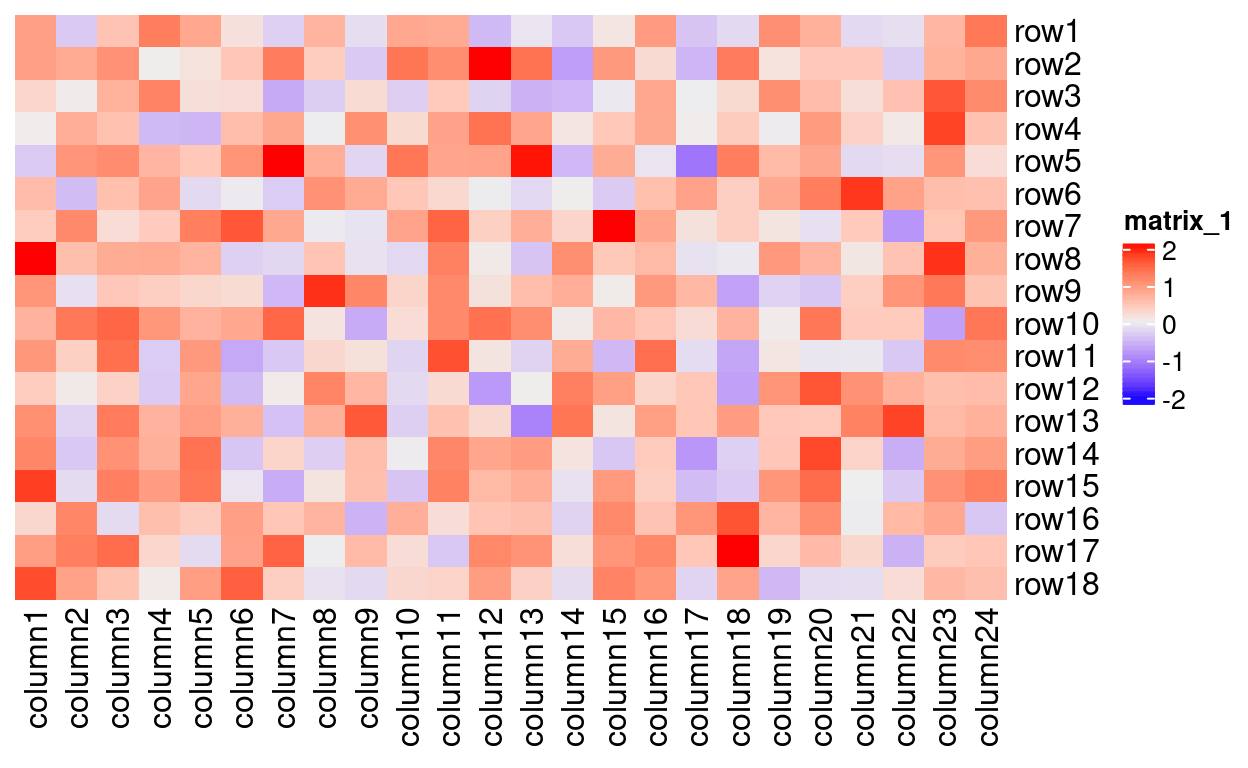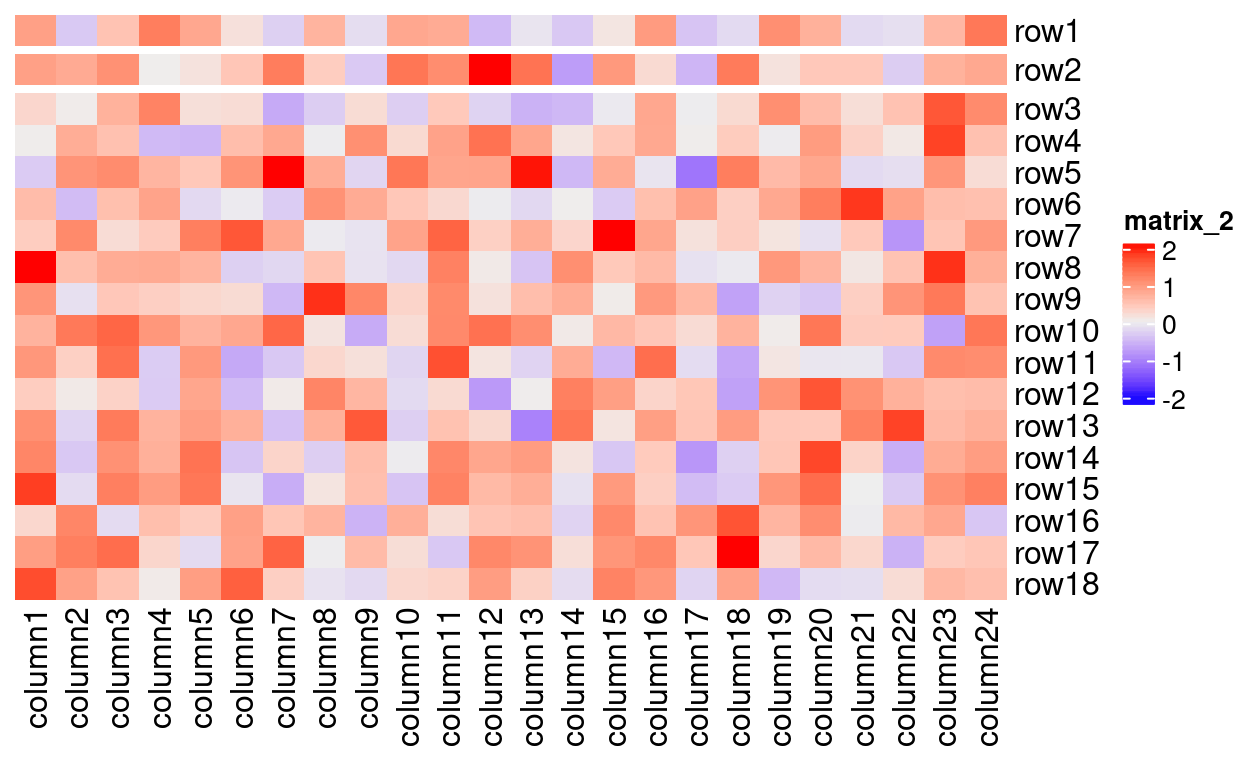Highlighting a Row of A ComplexHeatmap

A simple way to highlight or bring attention to a row or column in a ComplexHeatmap.

Robert M Flight true
03-26-2021

The ComplexHeatmap Bioconductor package has become my goto for visualizing sample-sample correlation heatmaps, which I use a lot. Recently, I had a report where I wanted to highlight a particular row of the heatmap. There is not an easy way that I could find to add something that wraps around a particular column. However, you can indicate that there is a grouping in the heatmap, and use that grouping to separate a sample or samples from the others.

Lets do an example:

library(ComplexHeatmap)
set.seed(123)
nr1 = 4; nr2 = 8; nr3 = 6; nr = nr1 + nr2 + nr3
nc1 = 6; nc2 = 8; nc3 = 10; nc = nc1 + nc2 + nc3
mat = cbind(rbind(matrix(rnorm(nr1*nc1, mean = 1,   sd = 0.5), nr = nr1),
matrix(rnorm(nr2*nc1, mean = 0,   sd = 0.5), nr = nr2),
matrix(rnorm(nr3*nc1, mean = 0,   sd = 0.5), nr = nr3)),
rbind(matrix(rnorm(nr1*nc2, mean = 0,   sd = 0.5), nr = nr1),
matrix(rnorm(nr2*nc2, mean = 1,   sd = 0.5), nr = nr2),
matrix(rnorm(nr3*nc2, mean = 0,   sd = 0.5), nr = nr3)),
rbind(matrix(rnorm(nr1*nc3, mean = 0.5, sd = 0.5), nr = nr1),
matrix(rnorm(nr2*nc3, mean = 0.5, sd = 0.5), nr = nr2),
matrix(rnorm(nr3*nc3, mean = 1,   sd = 0.5), nr = nr3))
)
mat = mat[sample(nr, nr), sample(nc, nc)] # random shuffle rows and columns
rownames(mat) = paste0("row", seq_len(nr))
colnames(mat) = paste0("column", seq_len(nc))

Heatmap(mat, cluster_rows = FALSE, cluster_columns = FALSE)Now, lets suppose we just want to highlight row2.

We create a data.frame with a factor to represent the grouping:

which_row2 = which(grepl("row2", rownames(mat)))
split = data.frame(x = c(rep("A", which_row2 - 1), "B",
rep("C", nrow(mat) - which_row2)))
Heatmap(mat, cluster_rows = FALSE, cluster_columns = FALSE,
row_split = split,
row_title = NULL)Voila! row2 is separated from the others to draw attention to it. It’s not perfect, but hopefully it’s useful to others. Note, that you can’t use clustering with this method. If you have actual dendrograms to display, this will fail, because ComplexHeatmap expects you to use a numeric argument to tell the cut height for dendrograms for splitting (Gu 2021c). Therefore, if you have dendrograms, reorder your columns and rows according to the dendrogram first and then add the splitting information and keep the clustering off.

Gu, Zuguang. 2021a. “ComplexHeatmap.” https://doi.org/10.18129/B9.bioc.ComplexHeatmap.
———. 2021b. “ComplexHeatmap Complete Reference.” https://jokergoo.github.io/ComplexHeatmap-reference/book/.
———. 2021c. “ComplexHeatmap Complete Reference.” https://jokergoo.github.io/ComplexHeatmap-reference/book/a-single-heatmap.html#heatmap-split.
Gu, Zuguang, Roland Eils, and Matthias Schlesner. 2016. “Complex Heatmaps Reveal Patterns and Correlations in Multidimensional Genomic Data.” Bioinformatics.

Corrections

If you see mistakes or want to suggest changes, please create an issue on the source repository.

Reuse

Text and figures are licensed under Creative Commons Attribution CC BY 4.0. Source code is available at https://github.com/rmflight/researchBlog_distill, unless otherwise noted. The figures that have been reused from other sources don't fall under this license and can be recognized by a note in their caption: "Figure from ...".